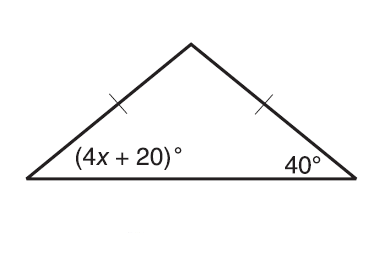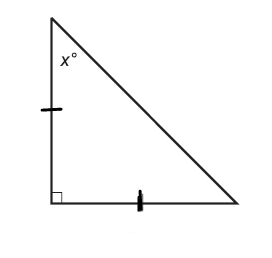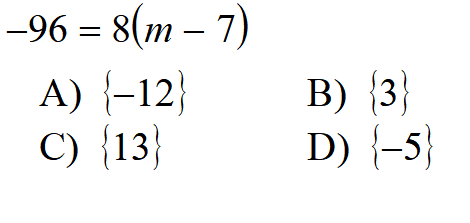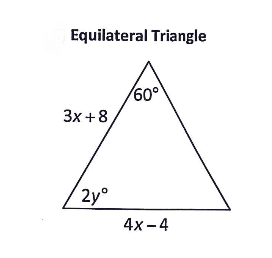Section 3 Skills Maint 3
solve *
1 pointIf 2 angles in a triangle add up to 124° then what is the value of the third angle? *
1 point
Last Name *
Each angle of an equilateral triangle is.... *
1 point
Which of the following cannot be the measure of three angles of a triangle? *
1 point
Period *
Can you have a triangle with two right angles? *
1 point
Find the measure of angle x. *
1 pointCan you have a triangle with all the three angles equal to 60º? *
1 point
solve *
1 pointCan you have a triangle with sides of 6, 6, and 12? *
1 point
In a right angle triangle, one of the angles is 53°. Which of the following can be another of theangles in the triangle? *
1 point
Find x in this triangle *
1 pointCan you have a triangle with two obtuse angles? *
1 point